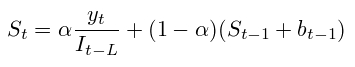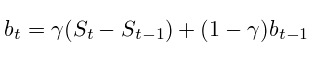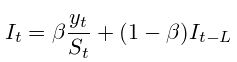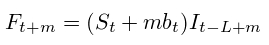# Triple Exponential Smoothing

On this page you will see a description and an example of a triple exponential smoothing.

If you skip the origins of this method, and move directly to the calculations, it is possible to express the triple exponential smoothing:

The general equation is as follows:Smoothing the trend is as follows:Smoothing seasonality as follows:The forecast can be expressed as:Where,

- A simple value within the range [0, 1]

y - simple observation

S - smoothed value monitoring

b - KOF. trends

I - seasonality index (year, month)

F - forecast by m periods ahead

t - index of current observations

﻿

Triple exponential smoothing
A number dynamics
Import data
For separation fields can use one from these characters: Tab, ";" or ",":
Standard error simple smoothing:
Standard error double smoothing:
Standard error triple smoothing:
Double exponential smoothing: